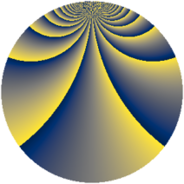# Properties

 Label 648.4.bbLevel $648$ Weight $4$ Character orbit 648.bb Rep. character $\chi_{648}(11,\cdot)$ Character field $\Q(\zeta_{54})$ Dimension $5796$ Sturm bound $432$

# Related objects

## Defining parameters

 Level: $$N$$ $$=$$ $$648 = 2^{3} \cdot 3^{4}$$ Weight: $$k$$ $$=$$ $$4$$ Character orbit: $$[\chi]$$ $$=$$ 648.bb (of order $$54$$ and degree $$18$$) Character conductor: $$\operatorname{cond}(\chi)$$ $$=$$ $$648$$ Character field: $$\Q(\zeta_{54})$$ Sturm bound: $$432$$

## Dimensions

The following table gives the dimensions of various subspaces of $$M_{4}(648, [\chi])$$.

Total New Old
Modular forms 5868 5868 0
Cusp forms 5796 5796 0
Eisenstein series 72 72 0

## Trace form

 $$5796q - 18q^{2} - 36q^{3} - 18q^{4} - 18q^{6} - 18q^{8} - 36q^{9} + O(q^{10})$$ $$5796q - 18q^{2} - 36q^{3} - 18q^{4} - 18q^{6} - 18q^{8} - 36q^{9} - 18q^{10} - 36q^{11} - 18q^{12} - 18q^{14} - 18q^{16} - 36q^{17} - 18q^{18} - 36q^{19} - 18q^{20} - 18q^{22} - 18q^{24} - 36q^{25} - 27q^{26} - 36q^{27} - 9q^{28} - 18q^{30} - 18q^{32} - 36q^{33} - 18q^{34} - 36q^{35} - 18q^{36} - 18q^{38} - 18q^{40} - 36q^{41} - 3573q^{42} - 36q^{43} + 2646q^{44} - 18q^{46} - 2295q^{48} - 36q^{49} + 4779q^{50} - 36q^{51} - 18q^{52} + 6660q^{54} - 5940q^{56} - 36q^{57} - 18q^{58} - 36q^{59} - 1305q^{60} - 1899q^{62} - 18q^{64} - 36q^{65} - 7434q^{66} - 36q^{67} + 7047q^{68} - 18q^{70} - 18q^{72} - 36q^{73} - 18q^{74} - 36q^{75} - 18q^{76} - 261q^{78} - 36q^{81} - 36q^{82} - 36q^{83} + 225q^{84} - 18q^{86} - 18q^{88} - 36q^{89} - 12555q^{90} - 36q^{91} - 11988q^{92} - 18q^{94} - 2943q^{96} - 36q^{97} + 2889q^{98} - 36q^{99} + O(q^{100})$$

## Decomposition of $$S_{4}^{\mathrm{new}}(648, [\chi])$$ into newform subspaces

The newforms in this space have not yet been added to the LMFDB.